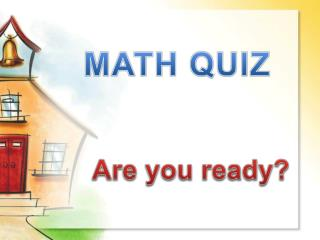DownloadDownload PresentationMATH QUIZ

# MATH QUIZ

Download Presentation## MATH QUIZ

- - - - - - - - - - - - - - - - - - - - - - - - - - - E N D - - - - - - - - - - - - - - - - - - - - - - - - - - -
##### Presentation Transcript

1. MATH QUIZ Are you ready?

2. Word Problem #1 Maria has three green apples and two red apples. Add all the apples. • Choose an answer. 3 + 1= 43 + 2 = 51 + 1 = 2

3. Try Again!

4. You got it! Next question

5. Word Problem #2 • Joe bought four pencils but gave one away to Ivan. • How many pencils does Joe have left? 4 – 1= 33 – 2 = 14 – 2 = 2

6. Try Again!

7. You got it! Next question

8. Word Problem #3 • Uriel has one soccer ball and two basketballs. • How many balls does Uriel have in all? 1 + 1 = 24 + 1 = 5 2 + 1 = 3

9. Try Again!

10. You got it! Next question

11. Money Problem #1 • How much money all together? 10 cents13 cents15 cents

12. Try Again!

13. You got it! Next question

14. Money Problem #2 • How much money in all? 25 cents50 cents75 cents

15. Try Again!

16. You got it! Next question

17. Money Problem #3 • What is the total? 40 cents42 cents 50 cents

18. Try Again!

19. You got it! Next question

20. Tens and Ones • How many tens and ones do you need to total 36? 3 tens and 6 ones 6 tens and 3 ones 4 tens and 2 ones

21. Try Again!

22. You got it! Next question

23. Tens and Ones • How many tens and ones do you need to make 15? 1 ten and 5 ones 3 tens and 2 ones 5 tens and 0 ones

24. Try Again!

25. You got it! Next question

26. Numbers from least to greatest • Order 12, 34, 23, 10 from least to greatest. • Which one is the correct answer? 23, 12, 10, 34 or 10, 12, 23, 34

27. Try Again!

28. You got it! Next question

29. Numbers from least to greatest • Order 6, 3, 1, 10 from least to greatest. • Which one is the correct answer? 1, 3, 6, 10 or 10, 6, 3, 1

30. Try Again!

31. You got it! Next question

32. Between Numbers • Choose the missing number between the two. 20, __, 40, 50, 60 70308090

33. Try Again!

34. You got it! Next question

35. Between Numbers Choose the missing numbers between the two 5, __, 7, 8, __, 10 3 and 1 or 6 and 9

36. Try Again!

37. You got it! Next question

38. Similar Shapes • Which shape is alike to ?

39. Try Again!

40. You got it! Next question

41. Similar Shapes • Which shape is similar to ?

42. Try Again!

43. You got it! Next question

44. Similar Shapes • Which shape is similar to ?

45. Try Again!

46. You got it! Next question

47. Congratulations YOU DID IT!